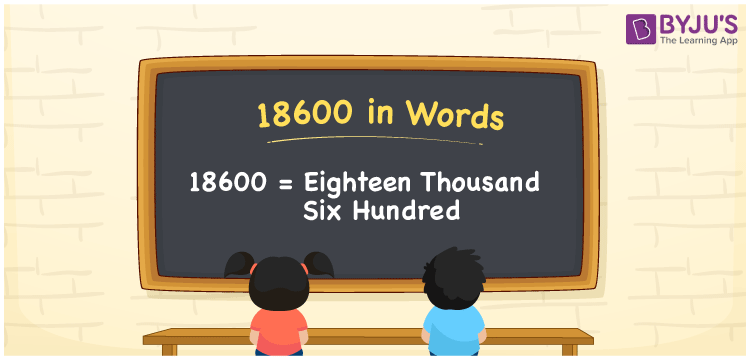# 18600 in Words

We can express 18600 in words as Eighteen Thousand Six Hundred. A conversion of 18600 to words is possible using a place value chart. If you have to write Rs.18600 on a cheque, then you can write, “Rupees Eighteen Thousand Six Hundred only”. 18600 is a cardinal number since it represents a specific quantity. In this article, learn more facts relying on the number 18600 along with learning how to write and read in English.

 18600 in words Eighteen Thousand Six Hundred Eighteen Thousand Six Hundred in numerical form 18600

## 18600 in English Words

Generally, In Mathematics, students write numbers in words using the English alphabet. Hence, 18600 in words is written as Eighteen Thousand Six Hundred.## How to Write 18600 in Words?

We know 18600 is a five-digit number. Hence, let us create a chart of 5 columns as shown below.

 Ten-Thousands Thousands Hundreds Tens Ones 1 8 6 0 0

Thus, the expanded form can be written as:

1 x Ten Thousand + 8 x Thousand + 6 x Hundred + 0 × Ten + 0 × One

= 1 x 10000 + 8 x 1000 + 6 x 100 + 0 x 10 + 0 x 1

= 10000 + 8000 + 600 + 0 + 0

= 10000 + 8000 + 600

= 18600

= Eighteen Thousand Six Hundred

Therefore, 18600 in words is written as Eighteen Thousand Six Hundred

Interesting way of writing 18600 in words

1 = One

18 = Eighteen

186 = One Hundred and Eighty-Six

1860 = One Thousand Eight Sixty

18600 = Eighteen Thousand Six Hundred

Hence, the word form of the number 18600 is Eighteen Thousand Six Hundred

18600 is a natural number that precedes 18601 and succeeds 18599

• 18600 in words – Eighteen Thousand Six Hundred
• Is 18600 an odd number? – No
• Is 18600 an even number? – Yes
• Is 18600 a perfect square number? – No
• Is 18600 a perfect cube number? – No
• Is 18600 a prime number? – No
• Is 18600 a composite number? – Yes

## Frequently Asked Questions on 18600 in Words

Q1

### How do you write 18600 in English?

We can write 18600 in English words as Eighteen Thousand Six Hundred.
Q2

### Write Eighteen Thousand Six Hundred in numbers.

Eighteen Thousand Six Hundred in numbers is 18600.
Q3

### Is 18600 a composite number?

Yes, 18600 is a composite number.